Home > MC2 > Chapter 11 > Lesson 11.2.4 > Problem11-68

11-68.
1. Solve for x. Homework Help ✎

1. 2(x + 18) = 10

2.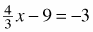3. 24 = 2(2x + 4)

4. 2(x – 4) = x – 7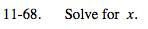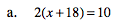Isolate the variable.

$\frac{2(x+18)}{2} = \frac{10}{2}$

$(x+18) = 5$

x = −13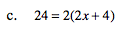See (a).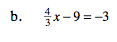See (a).

$\frac{18}{4}$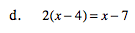See (a).

Distribute first.